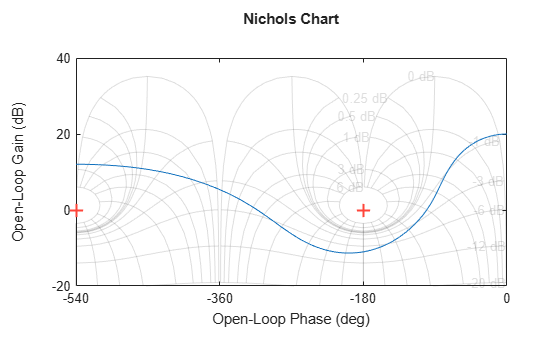Documentation

# nichols

Nichols chart of frequency response

## Syntax

```nichols(sys) nichols(sys,w) nichols(sys1,sys2,...,sysN) nichols(sys1,sys2,...,sysN,w) nichols(sys1,'PlotStyle1',...,sysN,'PlotStyleN') [mag,phase,w] = nichols(sys) [mag,phase] = nichols(sys,w) ```

## Description

`nichols` creates a Nichols chart of the frequency response. A Nichols chart displays the magnitude (in dB) plotted against the phase (in degrees) of the system response. Nichols charts are useful to analyze open- and closed-loop properties of SISO systems, but offer little insight into MIMO control loops. Use `ngrid` to superimpose a Nichols chart on an existing SISO Nichols chart.

`nichols(sys)` creates a Nichols chart of the dynamic system `sys`. This model can be continuous or discrete, SISO or MIMO. In the MIMO case, `nichols` produces an array of Nichols charts, each plot showing the response of one particular I/O channel. The frequency range and gridding are determined automatically based on the system poles and zeros.

`nichols(sys,w) ` specifies the frequency range or frequency points to be used for the chart. To focus on a particular frequency interval `[wmin,wmax]`, set `w = {wmin,wmax}`. To use particular frequency points, set `w` to the vector of desired frequencies. Use `logspace` to generate logarithmically spaced frequency vectors. Frequencies must be in `rad/TimeUnit`, where `TimeUnit` is the time units of the input dynamic system, specified in the `TimeUnit` property of `sys`.

`nichols(sys1,sys2,...,sysN)` or `nichols(sys1,sys2,...,sysN,w)` superimposes the Nichols charts of several models on a single figure. All systems must have the same number of inputs and outputs, but may otherwise be a mix of continuous- and discrete-time systems. You can also specify a distinctive color, linestyle, and/or marker for each system plot with the syntax `nichols(sys1,'PlotStyle1',...,sysN,'PlotStyleN')`.

See `bode` for an example.

```[mag,phase,w] = nichols(sys)``` or `[mag,phase] = nichols(sys,w)` returns the magnitude and phase (in degrees) of the frequency response at the frequencies `w` (in `rad/TimeUnit`). The outputs `mag` and `phase` are 3-D arrays similar to those produced by `bode` (see the `bode` reference page). They have dimensions

(number of outputs) × (number of inputs) × (length of w)

## Examples

collapse all

Plot the Nichols response with Nichols grid lines for the following system:

`$H\left(s\right)=\frac{-4{s}^{4}+48{s}^{3}-18{s}^{2}+250s+600}{{s}^{4}+30{s}^{3}+282{s}^{2}+525s+60}.$`

```H = tf([-4 48 -18 250 600],[1 30 282 525 60]); nichols(H) ngrid```The context menu for Nichols charts includes the Tight option under Zoom. You can use this option to clip unbounded branches of the Nichols chart.

## Tips

You can change the properties of your plot, for example the units. For information on the ways to change properties of your plots, see Ways to Customize Plots.

## Algorithms

See `bode`.